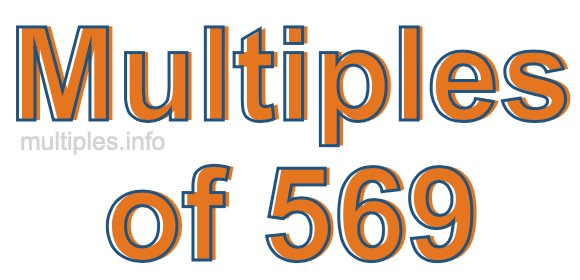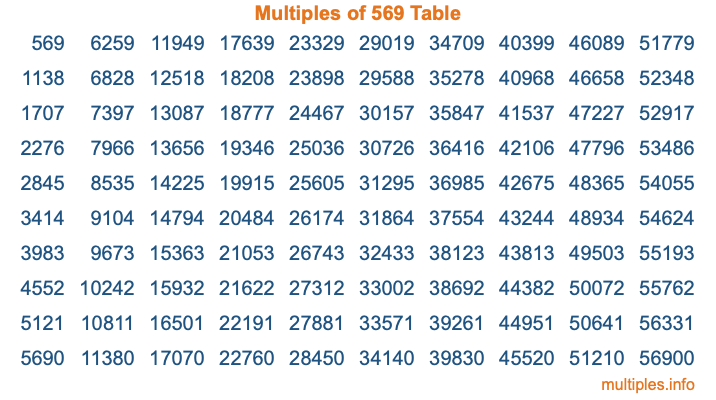Multiples of 569Welcome to the Multiples of 569 page. Here we will first teach you everything you will ever need to know about the multiples of 569, and then give you a study guide summary of everything we taught you to make sure you remember it all. Use this page to look up facts and learn information about the multiples of 569. This page will make you a multiples of five hundred sixty-nine expert!

Definition of Multiples of 569
Multiples of 569 are all the numbers that when divided by 569 equal an integer. Each of the multiples of 569 are called a multiple. A multiple of 569 is created by multiplying 569 by an integer.

Therefore, to create a list of multiples of 569, you start with 1 multiplied by 569, then 2 multiplied by 569, then 3 multiplied by 569, and so on for as long as you want. Thus, the list of the first five multiples of 569 is 569, 1138, 1707, 2276, and 2845. To see a larger list of multiples of 569, see the printable image of Multiples of 569 further down on this page. We also have a category where you can choose any nth multiple of 569.

Multiples of 569 Checker
The Multiples of 569 Checker below checks to see if any number of your choice is a multiple of 569. In other words, it checks to see if there is any number (integer) that when multiplied by 569 will equal your number. To do that, we divide your number by 569. If the the quotient is an integer, then your number is a multiple of 569.

Is  a multiple of 569?

Least Common Multiple of 569 and ...
A Least Common Multiple (LCM) is the lowest multiple that two or more numbers have in common. This is also called the smallest common multiple or lowest common multiple and is useful to know when you are adding our subtracting fractions. Enter one or more numbers below (569 is already entered) to find the LCM.

Check out our LCM Calculator if you need more details about the Least Common Multiple or if you need the LCM for different numbers for adding and subtraction fractions.

nth Multiple of 569
As we stated above, 569 is the first multiple of 569, 1138 is the second multiple of 569, 1707 is the third multiple of 569, and so on. Enter a number below to find the nth multiple of 569.

th multiple of 569

Multiples of 569 vs Factors of 569
569 is a multiple of 569 and a factor of 569, but that is where the similarities end. All postive multiples of 569 are 569 or greater than 569. All positive factors of 569 are 569 or less than 569.

Below is the beginning list of multiples of 569 and the factors of 569 so you can compare:

Multiples of 569: 569, 1138, 1707, 2276, 2845, etc.

Factors of 569: 1, 569

As you can see, the multiples of 569 are all the numbers that you can divide by 569 to get a whole number. The factors of 569, on the other hand, are all the whole numbers that you can multiply by another whole number to get 569.

It's also interesting to note that if a number (x) is a factor of 569, then 569 will also be a multiple of that number (x).

Multiples of 569 vs Divisors of 569
The divisors of 569 are all the integers that 569 can be divided by evenly. Below is a list of the divisors of 569.

Divisors of 569: 1, 569

The interesting thing to note here is that if you take any multiple of 569 and divide it by a divisor of 569, you will see that the quotient is an integer.

Multiples of 569 Table
Below is an image of the first 100 multiples of 569 in a table. The table is in chronological order, column by column. The first column has the first ten multiples of 569, the second column has the next ten multiples of 569, and so on.The Multiples of 569 Table is also referred to as the 569 Times Table or Times Table of 569. You are welcome to print out our table for your studies.

Negative Multiples of 569
Although not often discussed or needed in math, it is worth mentioning that you can make a list of negative multiples of 569 by multiplying 569 by -1, then by -2, then by -3, and so on, to get the following list of negative multiples of 569:

-569, -1138, -1707, -2276, -2845, etc.

Multiples of 569 Summary
Below is a summary of important Multiples of 569 facts that we have discussed on this page. To retain the knowledge on this page, we recommend that you read through the summary and explain to yourself or a study partner why they hold true.

There are an infinite number of multiples of 569.

A multiple of 569 divided by 569 will equal a whole number.

569 divided by a factor of 569 equals a divisor of 569.

The nth multiple of 569 is n times 569.

The largest factor of 569 is equal to the first positive multiple of 569.

569 is a multiple of every factor of 569.

569 is a multiple of 569.

A multiple of 569 divided by a divisor of 569 equals an integer.

569 divided by a divisor of 569 equals a factor of 569.

Any integer times 569 will equal a multiple of 569.

Multiples of a Number
Here you can get the multiples of another number, all with the same attention to detail as we did for multiples of 569 on this page.

Multiples of
Multiples of 570
Did you find our page about multiples of five hundred sixty-nine educational? Do you want more knowledge? Check out the multiples of the next number on our list!